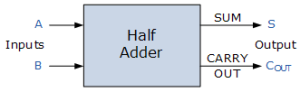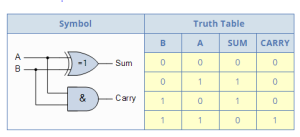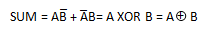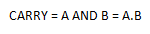Before discussing what is a half adder we need to know what is an adder first. Adder circuit is a combinational digital circuit that is used for adding two numbers.

A typical adder circuit produces a sum bit (denoted by S) and a carry bit (denoted by C) as the output.

Typically adders are realized  for adding binary numbers but they can be also realized  for adding other formats like BCD (binary coded decimal), XS-3 etc.

Besides addition, adder circuits can be used for a lot of other applications in digital electronics like address decoding, table index calculation etc.

Adder circuits are of two types:

A half adder is a logical circuit that performs an addition operation on two binary digits.

The half adder produces a sum (S) and a carry value (C) which are both binary digits.

The block diagram of a half adder is shown below in fig.1.Fig.1

Half Adder Truth Table with Carry-OutFig.2

From the truth table of the half adder we can see that the SUM (S) output is the result of the Exclusive-OR gate and the Carry-out (Cout) is the result of the AND gate.

Then the Boolean expression for a half adder is as follows:

For the SUM bit:For the CARRY bit:One major disadvantage of the Half Adder circuit when used as a binary adder, is that there is no provision for a “Carry-in” from the previous circuit when adding together multiple data bits.

The half adder can add only two input bits (A and B) and has nothing to do with the carry if there is any in the input. So if the input to a half adder have a carry, then it will be neglected it and adds only the A and B bits.

One simple way to overcome this problem is to use a Full Adder type binary adder circuit.